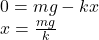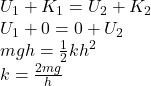## Kate, a bungee jumper, wants to jump off the edge of a bridge that spans a river below. Kate has a mass m, and the surface of the bridge is

Question

Kate, a bungee jumper, wants to jump off the edge of a bridge that spans a river below. Kate has a mass m, and the surface of the bridge is a height h above the water. The bungee cord, which has length L when unstretched, will first straighten and then stretch as Kate falls. Assume the following: The bungee cord behaves as an ideal spring once it begins to stretch, with spring constant k. Kate doesn’t actually jump but simply steps off the edge of the bridge and falls straight downward. Kate’s height is negligible compared to the length of the bungee cord. Hence, she can be treated as a point particle. Use g for the magnitude of the acceleration due to gravity.
(a) How far below the bridge will Kate eventually be hanging, once she stops oscillating and comes finally to rest? Assume that she doesn’t touch the water.
(b) If Kate just touches the surface of the river on her first downward trip (i.e. before the first bounce), what is the spring constant k?

in progress 0
5 months 2021-09-04T11:11:32+00:00 1 Answers 63 views 0

(a) x = mg/k

(b) k = 2mg/h

Explanation:

(a) Kate will hang without oscillating when the equilibrium condition is satisfied.

At equilibrium, the net force on Kate will be equal to zero.

Therefore,(b) If Kate touches the surface of the river on her first downward trip, then by the conservation of energy we can find the spring constant: